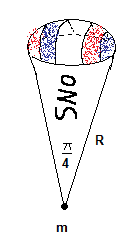# Force of Gravity on the tip of a giant snowcone

#### m00npirate

1. The problem statement, all variables and given/known data
I'm studying for a physics exam and made up the following problem to practice. Since I made it up there is no way to check my answer and I would greatly appreciate any and all feedback.

Find the force of gravity exerted on a point mass m at the tip of a snowcone (portion of a ball cut out by a cone where the radius of the sphere = the length of the side of the cone) with constant density ϱ and radius R. The internal angle of the cone is pi/4 in both directions.2. Relevant equations
F = -GmM(r)/r^2

3. The attempt at a solution
I used spherical coordinates
M(r)=ϱV(r)

M(r)=$$\frac{-\rho}{3}r^{3}\theta\cos\phi$$

$$dM(r)=r^{2}\sin\phi dr d\phi d\theta$$

$$F=\int_0^{\pi/4}\int_0^{\pi/4}\int_0^R \frac{-Gm\rho r^{2}}{r^{2}} \sin\phi dr d\phi d\theta$$

$$F=-\frac{\pi}{4} Gm\rho\int_0^{\pi/4} \sin\phi d\phi \int_0^R dr$$

$$F=-\frac{\pi}{4} Gm\rho R(\cos0-\cos\frac{\pi}{4})$$

$$F=-\frac{\pi}{8} (2-\sqrt{2})Gm\rho R$$

Also, I can't think of any way to find F(d) where d is the distance from the point of the cone. It might just be wishful thinking though.

Last edited:

#### LowlyPion

Homework Helper
I might consider solving by superposition.

The sum of the conical solid and the spherical one.

For the cone you can sum the individual disks of dm = ϱ*a(x)dx and then add the spherical section calculated from the distance of the cone height.

I guess I don't see the need to use the spherical coordinates.

### The Physics Forums Way

We Value Quality
• Topics based on mainstream science
• Proper English grammar and spelling
We Value Civility
• Positive and compassionate attitudes
• Patience while debating
We Value Productivity
• Disciplined to remain on-topic
• Recognition of own weaknesses
• Solo and co-op problem solving